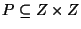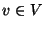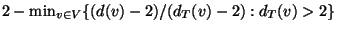Next: MAXIMUM LEAF SPANNING TREE Up: Spanning Trees Previous: MINIMUM DEGREE SPANNING TREE   Index

### MINIMUM GEOMETRIC 3-DEGREE SPANNING TREE

• INSTANCE: Setof points in the plane.

• SOLUTION: A spanning tree T for P in which no vertex has degree larger than 3.

• MEASURE: The total weight of the spanning tree, i.e.,, where d(u,v) is the Euclidean distance between u and v.

• Good News: Admits a PTAS .

• Comment: The 4-degree spanning tree problem also admits a PTAS, but the NP-hardness of the problem is open . The 5-degree problem is polynomial-time solvable. In d-dimensional Euclidean space forthe 3-degree spanning tree problem is approximable within 5/3. .

The generalization to a graph with metric weight function and for each vertexÝa degree bound d(v) is called MINIMUM BOUNDED DEGREE SPANNING TREE and is approximable within, whereis the initial degree of v .

Viggo Kann
2000-03-20